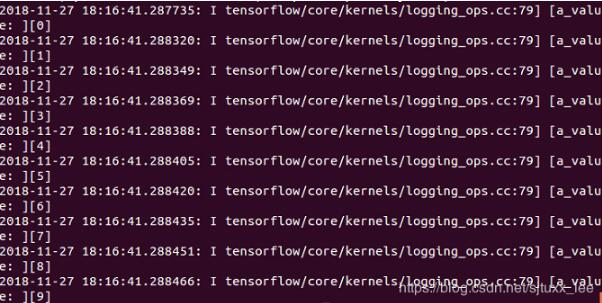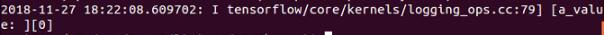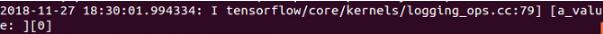# tensorflow实现在函数中用tf.Print输出中间值

tensorflow由于其基于静态图的模式，导致写代码的时候很难调试，除了用官方的调试工具外

tensorflow由于其基于静态图的模式，导致写代码的时候很难调试，除了用官方的调试工具外，最直接的方法就是把中间结果输出出来查看，然而，直接用print函数只能输出tensor变量的形状，而不是数值，想要输出tensor的具体数值需要用tf.Print函数。网上有很多关于这个函数使用方法的说明，这里简要介绍：

``````
Print(
input_,
data,
message=None,
first_n=None,
summarize=None,
name=None
)``````

input_：通过这个操作的张量。 （流入的数据流）

data：计算 op 时要打印的张量列表。（用[ ]引起来的一串需要打印的东西，用逗号隔开）

message：一个字符串，错误消息的前缀。

first_n：只记录 first_n 次数。负数日志，这是默认的。

summarize：只打印每个张量的固定数目的条目。如果没有，则每个输入张量最多打印3个元素。

name：操作的名称（可选）

``````
import tensorflow as tf
import os

os.environ["CUDA_VISIBLE_DEVICES"] = "0"

def test():
a=tf.constant(0)
for i in range(10):
a_print = tf.Print(a,['a_value: ',a])
a=a_print+1
return a

if __name__=='__main__':
with tf.Session() as sess:
sess.run(test())
``````a_print可以理解为在图中新增了一个节点，在后续代码中当有别的变量使用了a_print时（如上例a=a_print+1），就会有数据从a_print节点上流过，就会输出值，而究竟会输出几次值呢？这其实并不是看下文中a_print被使用了几次，而是看数据流要从该节点上流经几次，可以理解为a_print这个op被“定义”了几次。

``````
def test():
a=tf.constant(0)
a_print = tf.Print(a,['a_value: ',a])
for i in range(10):
a=a_print+1
return a

if __name__=='__main__':
with tf.Session() as sess:
sess.run(test())````````````
def test():
a=tf.constant(0)
a_print = tf.Print(a,['a_value: ',a])
for i in range(10):
a_print=a_print+1
return a

if __name__=='__main__':
with tf.Session() as sess:
sess.run(test())``````

``````
def test():
a=tf.constant(0)
a_print = tf.Print(a,['a_value: ',a])
for i in range(10):
a_print=a_print+1
return a_print

if __name__=='__main__':
with tf.Session() as sess:
sess.run(test())``````